## At the bottom of its path, the ball strikes a 2.30 kg steel block initially at rest on a frictionless surface. The collision is elastic. Fin

Question

At the bottom of its path, the ball strikes a 2.30 kg steel block initially at rest on a frictionless surface. The collision is elastic. Find (a) the speed of the ball and (b) the speed of the block, both just after the collision.

in progress 0
2 weeks 2021-09-04T20:36:54+00:00 1 Answers 0 views 0

(a). The speed of the ball after collision is 2.01 m/s.

(b). The speed of the block after collision 1.11 m/s.

Explanation:

Suppose, A steel ball of mass 0.500 kg is fastened to a cord that is 50.0 cm long and fixed at the far end. The ball is then released when the cord is horizontal.

Given that,

Mass of steel block = 2.30 kg

Mass of ball = 0.500 kg

Length of cord = 50.0 cm

We need to calculate the initial speed of the ball

Using conservation of energy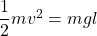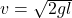Put the value into the formula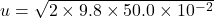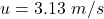The initial speed of the ball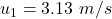The initial speed of the block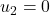(a). We need to calculate the speed of the ball after collision

Using formula of collision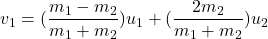Put the value into the formula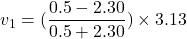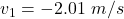Negative sign shows the opposite direction of initial direction.

(b). We need to calculate the speed of the block after collision

Using formula of collision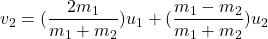Put the value into the formula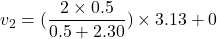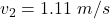Hence, (a). The speed of the ball after collision is 2.01 m/s.

(b). The speed of the block after collision 1.11 m/s.